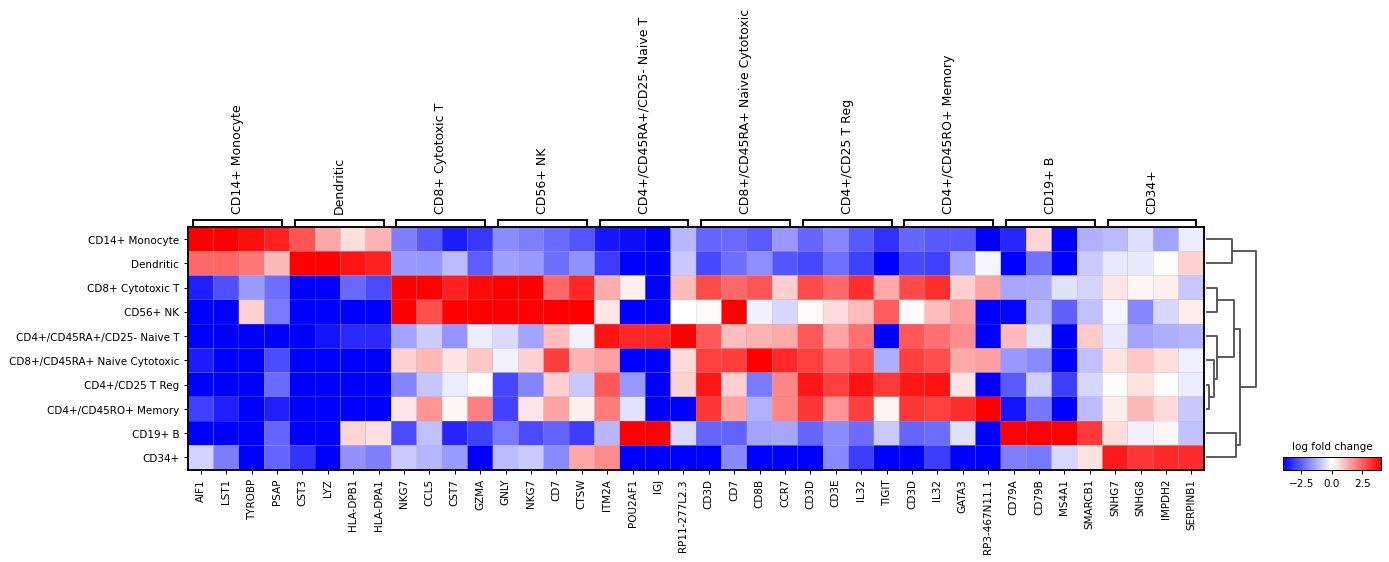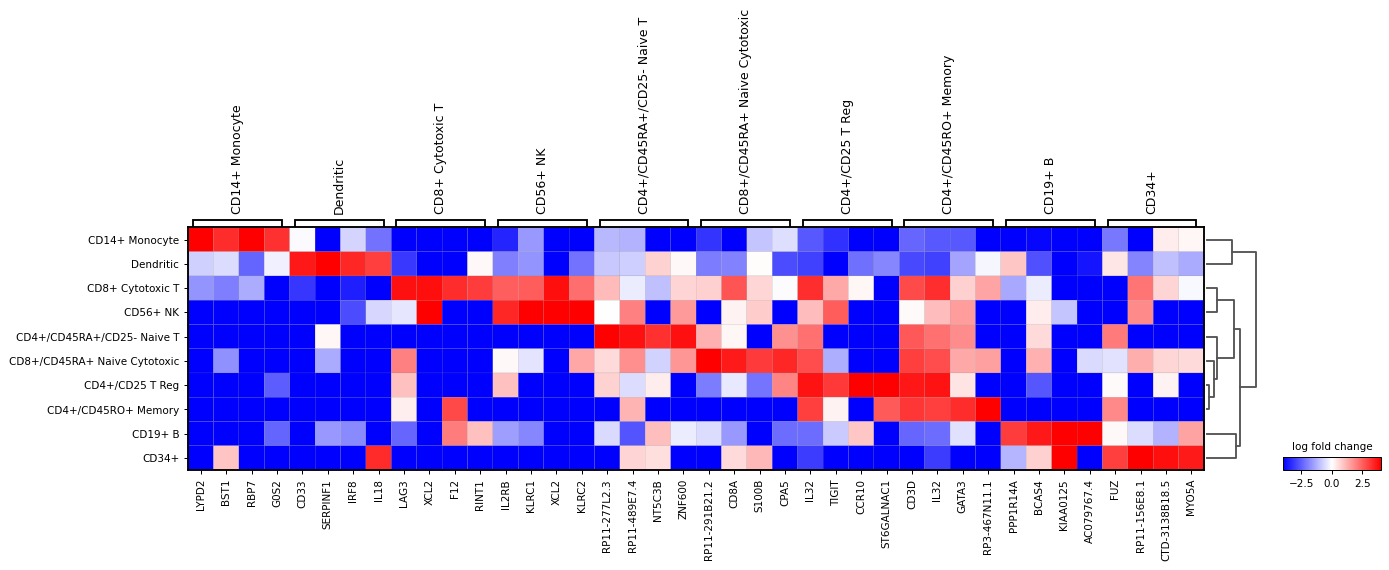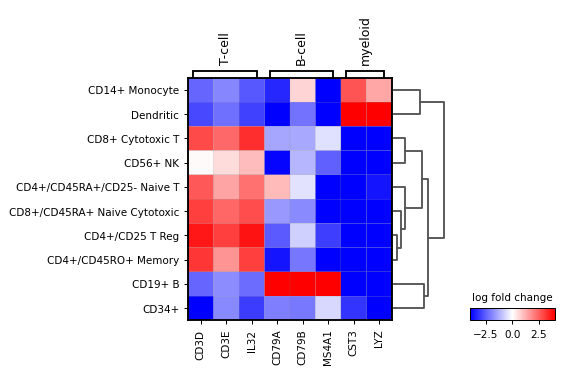# scanpy.pl.rank_genes_groups_matrixplot¶

scanpy.pl.rank_genes_groups_matrixplot(adata, groups=None, n_genes=None, groupby=None, values_to_plot=None, var_names=None, gene_symbols=None, min_logfoldchange=None, key=None, show=None, save=None, return_fig=False, **kwds)

Plot ranking of genes using matrixplot plot (see `matrixplot()`)

Parameters
adata : `AnnData``AnnData`

Annotated data matrix.

groups : (default: `None`)

The groups for which to show the gene ranking.

n_genes : (default: `None`)

Number of genes to show. This can be a negative number to show for example the down regulated genes. eg: num_genes=-10. Is ignored if `gene_names` is passed.

gene_symbols : (default: `None`)

Column name in `.var` DataFrame that stores gene symbols. By default `var_names` refer to the index column of the `.var` DataFrame. Setting this option allows alternative names to be used.

groupby : (default: `None`)

The key of the observation grouping to consider. By default, the groupby is chosen from the rank genes groups parameter but other groupby options can be used. It is expected that groupby is a categorical. If groupby is not a categorical observation, it would be subdivided into `num_categories` (see `dotplot()`).

min_logfoldchange : (default: `None`)

Value to filter genes in groups if their logfoldchange is less than the min_logfoldchange

key : (default: `None`)

Key used to store the ranking results in `adata.uns`.

values_to_plot : {‘scores’, ‘logfoldchanges’, ‘pvals’, ‘pvals_adj’, ‘log10_pvals’, ‘log10_pvals_adj’} | `None``Optional`[`Literal`[‘scores’, ‘logfoldchanges’, ‘pvals’, ‘pvals_adj’, ‘log10_pvals’, ‘log10_pvals_adj’]] (default: `None`)

Instead of the mean gene value, plot the values computed by `sc.rank_genes_groups`. The options are: [‘scores’, ‘logfoldchanges’, ‘pvals’, ‘pvals_adj’, ‘log10_pvals’, ‘log10_pvals_adj’]. When plotting logfoldchanges a divergent colormap is recommended. See examples below.

var_names : (default: `None`)

Genes to plot. Sometimes is useful to pass a specific list of var names (e.g. genes) to check their fold changes or p-values, instead of the top/bottom genes. The var_names could be a dictionary or a list as in `dotplot()` or `matrixplot()`. See examples below.

show : (default: `None`)

Show the plot, do not return axis.

save : (default: `None`)

If `True` or a `str`, save the figure. A string is appended to the default filename. Infer the filetype if ending on {`'.pdf'`, `'.png'`, `'.svg'`}.

ax

A matplotlib axes object. Only works if plotting a single component.

return_fig : (default: `False`)

Returns `MatrixPlot` object. Useful for fine-tuning the plot. Takes precedence over `show=False`.

**kwds

Are passed to `matrixplot()`.

Returns

If `return_fig` is `True`, returns a `MatrixPlot` object, else if `show` is false, return axes dict

Examples

```import scanpy as sc
```

Plot `logfoldchanges` instead of gene expression. In this case a diverging colormap like `bwr` or `seismic` works better. To center the colormap in zero, the minimum and maximum values to plot are set to -4 and 4 respectively. Also, only genes with a log fold change of 3 or more are shown.

```sc.pl.rank_genes_groups_matrixplot(
n_genes=4,
values_to_plot="logfoldchanges",
cmap='bwr',
vmin=-4,
vmax=4,
min_logfoldchange=3,
colorbar_title='log fold change',
)
```Also, the last genes can be plotted. This can be useful to identify genes that are lowly expressed in a group. For this `n_genes=-4` is used

```sc.pl.rank_genes_groups_matrixplot(
n_genes=-4,
values_to_plot="logfoldchanges",
cmap='bwr',
vmin=-4,
vmax=4,
min_logfoldchange=3,
colorbar_title='log fold change',
)
```A list specific genes can be given to check their log fold change. If a dictionary, the dictionary keys will be added as labels in the plot.

```var_names = {"T-cell": ['CD3D', 'CD3E', 'IL32'],
'B-cell': ['CD79A', 'CD79B', 'MS4A1'],
'myeloid': ['CST3', 'LYZ'] }
sc.pl.rank_genes_groups_matrixplot(